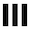##dwitter.net

+ New dweet
`function u(t) {`
```} // 122/140 ```

Please log in (or register) to post a dweet (copy-paste the code somewhere safe to save it meanwhile)

``` u(t) is called 60 times per second. t: Elapsed time in seconds. S: Shorthand for Math.sin. C: Shorthand for Math.cos. T: Shorthand for Math.tan. R: Function that generates rgba-strings, usage ex.: R(255, 255, 255, 0.5) c: A 1920x1080 canvas. x: A 2D context for that canvas. ```
`function u(t) {`
```}// 140/140 ```
``` u(t) is called 60 times per second. t: elapsed time in seconds. c: A 1920x1080 canvas. x: A 2D context for that canvas. S: Math.sin C: Math.cos T: Math.tan R: Generates rgba-strings, ex.: R(255, 255, 255, 0.5) ```